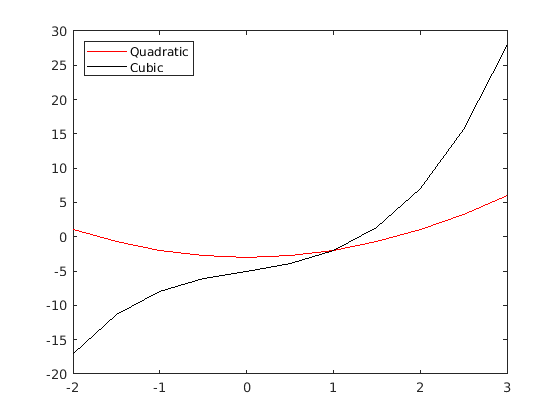## Week 1 Coding Lecture 4: Plotting

In this lecture, we will go over how to create graphs in MATLAB. As an example, let's start by trying to plot the curve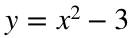.
The method MATLAB uses to create graphs is very similar to the way you were probably taught in your first algebra class. You first make a list of x-values, then you plug each x-value into your formula to find a corresponding y-value, then you plot each of these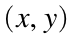pairs as a point on your graph. Once you plot enough points, you can then connect the dots in some way to make the picture look like a smooth curve.
To do this in MATLAB, you first need to create a vector that will hold all of your x-values. You can use either a row or column vector, but it is a good idea to be consistent. I will use row vectors throughout this document because they take less space to display, but it is perfectly fine to use all column vectors instead. Unless you have a specific reason not to, it is common to just create evenly spaced x-values using either the colon operator or the linspace function. For example, if we wanted to plot our curve between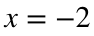and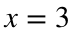, then we could use the code
x = linspace(-2, 3, 10)
x = 1×10
-2.0000 -1.4444 -0.8889 -0.3333 0.2222 0.7778 1.3333 1.8889 2.4444 3.0000
to make ten evenly spaced x-values. Alternatively, we could use the code
x = -2:0.5:3
x = 1×11
-2.0000 -1.5000 -1.0000 -0.5000 0 0.5000 1.0000 1.5000 2.0000 2.5000 3.0000
to make x-values spaced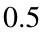apart. The choice of x-values is up to you, but we will soon see that using more values will usually make your graph look nicer.
Next, you need to plug each of your x-values into the formula for whatever function you are trying to plot. In our case, we want to plot, so we need to square each entry of x and then subtract 3 from each entry. This is a place where elementwise operations are extremely useful. We can use the code
y = x.^2 - 3
y = 1×11
1.0000 -0.7500 -2.0000 -2.7500 -3.0000 -2.7500 -2.0000 -0.7500 1.0000 3.2500 6.0000
Notice that we had to use a .^ instead of just ^ because we want to square each element of x rather than use matrix multiplication. Typically, when you are plotting you will only want elementwise operations, so all multiplication, division and exponents will need dots (i.e., .* and ./ and .^).
Now we have everything we need to make a graph. We do so using the plot command. The basic syntax is plot(vector_of_x_values, vector_of_y_values)
plot(x, y)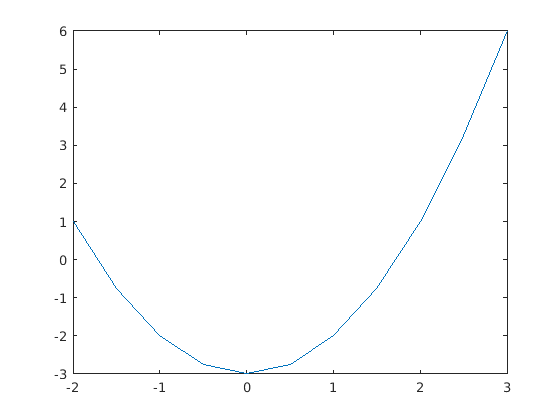If you are working in a live script like this (a .mlx file) then the graph will show up in the document. Otherwise, it should pop up in its own window.
Notice that this isn't really a smooth curve. Matlab has plotted the 11 points that we specified with the vectors x and y and then just connected the dots with straight lines.

### Line Properties

#### Color

We can modify the appearance of this graph in many different ways. For example, we might want to change the color of the line. There are two ways to do so. First, MATLAB has several shortcuts for colors. For example, 'k' stands for black, 'b' stands for blue and 'r' stands for red. (You can find a list of all of these shortcuts by searching for "LineSpec" in the MATLAB documentation.) If we wanted a red curve, we could use
plot(x, y, 'r')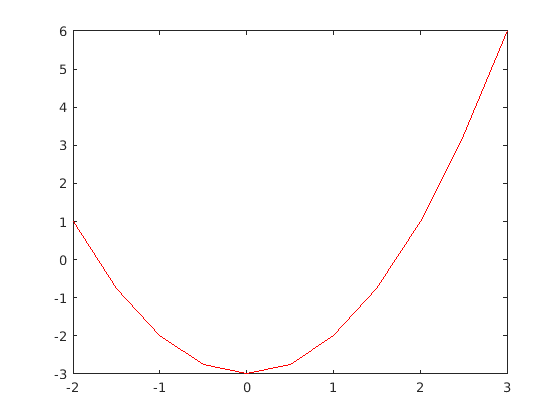(Note that the quotation marks are necessary. You can either use single quotes, like 'r', or double quotes, like "r", but you can't just use r.) There are only a few pre-defined colors, but you can use any color you want by specifying the RGB value or hex color code. (Any reasonable paint program or online color picker will give you these values; you don't have to remember them.) As an example, the hex code #7E2F8E stands for a shade of purple. You can specify this color with
plot(x, y, 'Color', '#7E2F8E')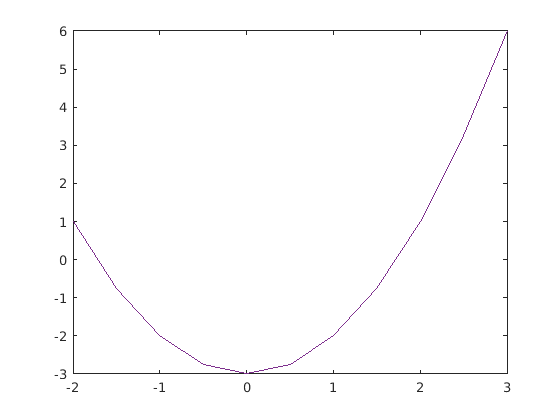Again, notice the quotation marks. They can be either single or double quotes, but they are not optional.

#### Style

You can also change the line style (for instance, dotted lines, dashed lines, etc.) There are shortcut symbols for all of these. You do not need to memorize the symbols - you can look them up in the MATLAB documentation for plot whenever necessary. As an example, the shortcut ':' stands for dotted line, so you can use
plot(x, y, ':')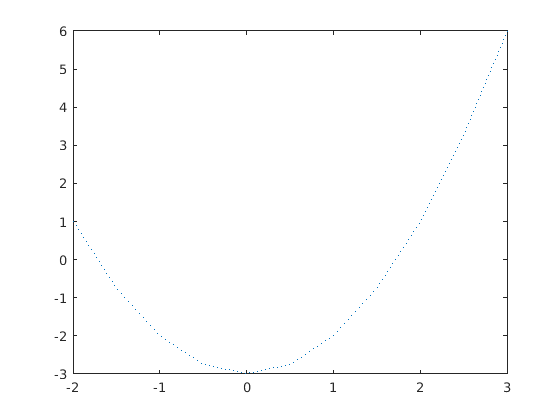Shortcuts can be combined, so you could make a red dotted line with
plot(x, y, 'r:')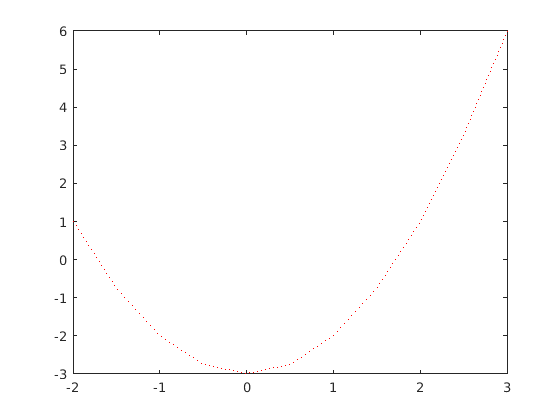You can also specify these with a more verbose syntax like so:
plot(x, y, 'LineStyle', ':')This is not as useful as with colors, because all of the line styles have a shortcut.

#### Marker Shape

You can also specify the shape of the markers (i.e., the shape MATLAB puts at each data point). As with the line style, there are shortcuts for all of the available shapes. One particularly useful one is 'o', which makes open circles at each point. For instance,
plot(x, y, 'o')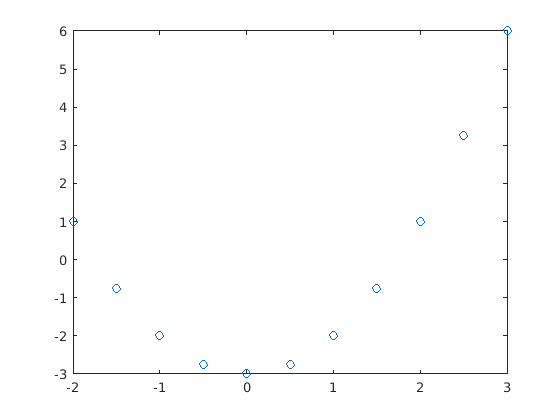You can combine any of these shortcuts. For instance, the following code makes a black dotted line with open circles at each point:
plot(x, y, 'k:o')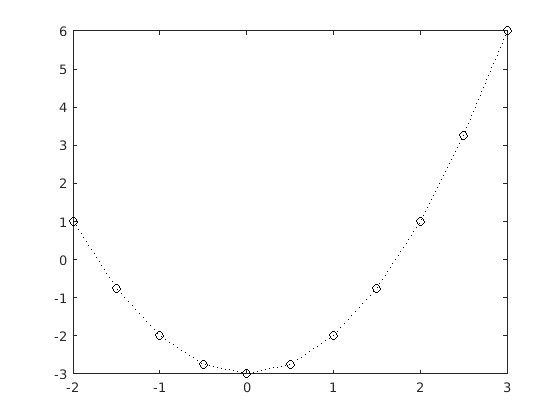There is also a more verbose syntax:
plot(x, y, 'Marker', 'o')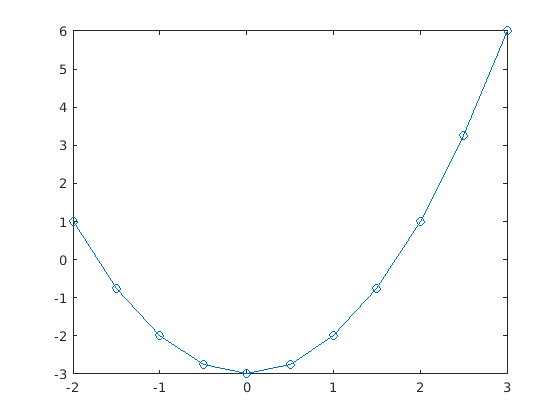There are many other options that you can modify, but the others don't come with shortcuts. They can all be modified with the long version of the syntax above. As one example, you can change the width of the line with the 'LineWidth' option like so:
plot(x, y, 'LineWidth', 10)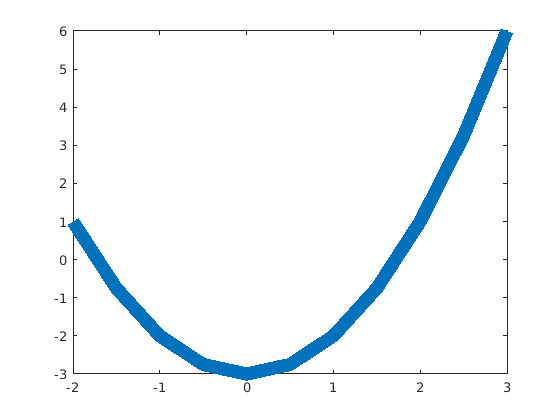You can combine as many options as you want in this way. For example,
plot(x, y, 'Color', 'r', 'LineStyle', '--', 'LineWidth', 3, 'Marker', '+')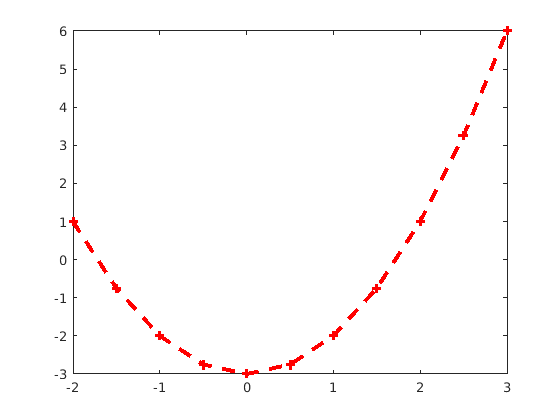There are many more options. I encourage you to look through the documentation for plot and try different things out. I will not give you exact specifications for plots in this class, but I will expect you to choose options that make your graph look decent. For instance, if you are plotting more than one curve on the same graph (which we will do in a moment), then you should make each one a different color.

### Labels and Titles

You can also customize the rest of the graph as well. We will only look at a few of the simplest options here, but I strongly encourage you to explore the MATLAB documentation and see how to change other features.
Two of the most useful things you can change are the axis labels and titles. You can do so with the commands xlabel, ylabel and title. Each takes one input: a string of text (encased in quotation marks) that will be displayed in the appropriate place. For instance,
plot(x, y, 'r')
xlabel("x-axis")
ylabel("y-axis")
title("Graph of a parabola")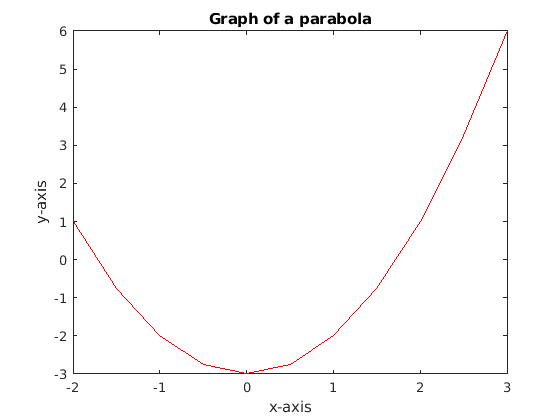For the rest of this class, every plot you make should have axis labels and a title, even if the labels are just "x" and "y".

### Plotting Multiple Curves

Very often, we will want to plot more than one curve. Unfortunately, if you try to use two plot commands in a script or in the command window, only one of the curves will appear. As an example, let's try to plotin red and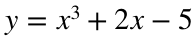in black. The obvious try would be
x = -2:0.5:3;
y = x.^2 - 3;
z = x.^3 + 2*x - 5;
plot(x, y, 'r')plot(x, z, 'k')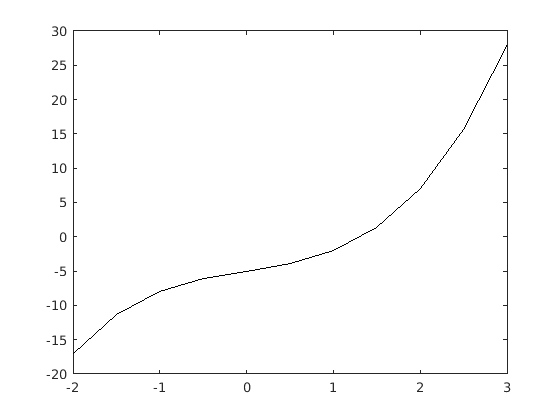In an .mlx file like this, both plots appear on separate figures, but if you run the same code in a script you will only see the second graph. There are two ways to fix this issue. The easiest is to combine both curves into a single plot command. The syntax is plot(x1, y1, as_many_specifications_as_you_want, x2, y2, other_specifications). For example,
plot(x, y, 'r', x, z, 'k')
This is nice if you have all of the data for both graphs in the same place, but often there will be many lines of code between where you calculated y and where you calculated z. To fix this, you can tell MATLAB to put everything in the same figure with the command hold on. It is good practice to turn off this feature once you are done plotting, so after all of the plot commands you should include the command hold off. For instance,
hold on
plot(x, y, 'r')
plot(x, z, 'k')
hold off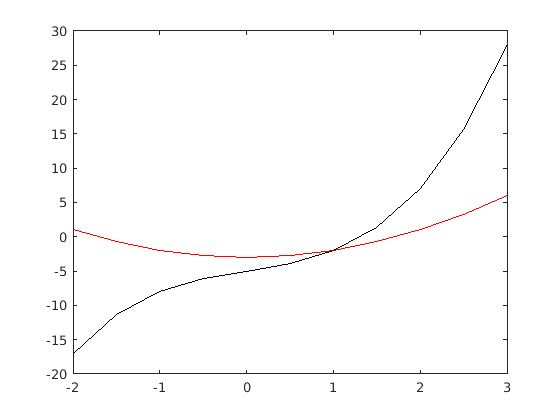You can put as much code (including as many plot statements as you want) in between the hold on and hold off statements.
If you include multiple curves on the same plot, it is always good practice to add a legend so that readers will be able to understand your graph. You can do so with the legend command. The syntax is legend(label1, label2, ...)
where each label is encased in quotation marks. The labels should go in the same order that you made the plots. For example,
hold on
plot(x, y, 'r')
plot(x, z, 'k')
hold off
MATLAB is not always great about placing the legend. For instance, in the previous figure the legend covered up part of the graph, even though there was plenty of open space available. You can manually adjust the position with the following syntax:
hold on
plot(x, y, 'r')
plot(x, z, 'k')
hold off